# Maths - Cube Rotation

As an example of a permutation group (for an introduction to permutation groups see this page) we will look at a finite subset of the 3D rotation group SO(3), so we will look at all the rotation transforms of a cube that map it to itself. For information about 3D rotations see this page.

We can study cube rotation using the various algebras associated with 3D rotations such as:

but the aim on this page is to separate the properties of this rotation in a way that is not dependant on the properties of any specific algebra and instead brings out the higher level symmetries.

Lets take as an example the rotation of a cube in ways that does not change its shape (i.e. multiples of 90° rotations about x, y or z) although we will keep track of the rotations possibly by markings on the faces of the cube. We could have chosen other platonic solids, other then a cube, such as a dodecahedron. These other platonic solids are described on this page.

There are a number of ways to analyise this, one way is to number the vertices and track the effect of each rotation on these vertices.So a 90° rotation about each of the x, y or z axis could be defined as follows (as a cycle decomposition described on this page)

x = rotation about x = (1, 4, 8, 5) (2, 3, 7, 6)
y =rotation about y = (1, 5, 6, 2) (4, 8, 7, 3)
z = rotation about z = (1, 2, 3, 4) (5, 6, 7, 8)

We now need to work out all the permutations of these rotations.

There are 24 made up of 1 identity element, 9 rotations about opposite faces, 8 rotations about opposite vertices and 6 rotations about opposite lines.

number of rotations
rotate about opposite faces9

(3 for each pair of faces)

rotate about opposite vertices8

(2 for each pair of vertices)

rotate about opposite lines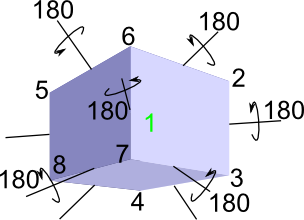6

(one for each pair of lines)

This gives 9 + 8 + 6 = 23 possible rotations of the cube, plus the identity element (leave it where it is giving 24 possible rotations in total.

There is a quicker way to discover that there are 24 rotations. If we realise that there is a one to one correspondence between rotations and possible orientations of the cube, then all we have to do is count the possible orientations, for instance we could:

• realise that there are 6 faces and so we can place it on each face in turn in 4 possible ways giving 6*4 = 24 possible orientations.
• realise that there are 8 vertices and so we can place it on each vertex in a given position in 3 possible ways giving 8*3 = 24 possible orientations.

### Permutation Group of Cube

This has 24 possible rotations, we can generate these by starting with the identity element and the 90° rotations about the x, y and z axis, then by combining these in different sequences we can generate all 24 permutations. In fact, as we shall see below, we only need to start with 2 permutations to generate all possible rotations.

   permutation cycle notation identity (0°) about x (90°) about y (90°) about z (90°)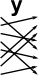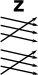(1, 4, 8, 5) (2, 3, 7, 6) (1, 5, 6, 2) (4, 8, 7, 3) (1, 2, 3, 4) (5, 6, 7, 8)

We will denote these permutations by the letters i ,x ,y and z. The composition of x and y (y o x) will be denoted xy:#### o#### =To be consistent with other literature on this, we need to reverse the order of the functions, that is the right hand function is applied first then the left hand function. So, for example, xy = (y o x).

We can draw up a complete table of the composition operation which defines this example completely. It is a bit of a drudge to work out all these permutations by hand so I have written a program to do this automatically - This is still work in progress - see this page.

### Generating rotations from i and x

In order to try out the program with a simple example, I supplied the program with 2 permutations ('i' and 'x') to start it going and it generated two more to complete the group:

   permutation cycle notation identity (0°) about x (90°) about x (180°) about x (270°)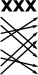(1, 4, 8, 5) (2, 3, 7, 6) (8 1)(7 2)(6 3)(5 4) (5 8 4 1)(6 7 3 2)

So from the generators: 'i' and 'x' the program has generated two other permutations 'xx' (apply x twice) and 'xxx' (apply x three times).

The complete multiplication (Cayley) table for this group is then:

 a*b b.i b.x b.xx b.xxx a.i i x xx xxx a.x x xx xxx i a.xx xx xxx i x a.xxx xxx i x xx

### Generating rotations from i, x and y

This time I supplied the program with 3 permutations ('i' , 'x' and 'y') to start it going and it generated 21 more to complete the group of 24:

permutation cycle notation permutation cycle notation(1, 4, 8, 5) (2, 3, 7, 6) (1, 5, 6, 2) (4, 8, 7, 3) (8 1)(7 2)(6 3)(5 4)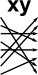(8 6 1)(4 7 2)(3)(5) (1)(4 5 2)(8 6 3)(7) (6 1)(5 2)(8 3)(7 4) (5 8 4 1)(6 7 3 2)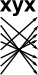(7 1)(3 2)(6 4)(8 5) (5 1)(8 2)(7 3)(6 4) (7 1)(8 2)(4 3)(6 5) (4 1)(8 2)(5 3)(7 6)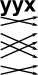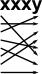(2 1)(5 3)(6 4)(8 7) (2 6 5 1)(7 8 4 3) (6 3 1)(2)(5 7 4)(8) (6 8 1)(7 4 2)(3)(5)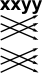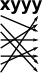(3 1)(4 2)(7 5)(8 6) (1)(5 4 2)(6 8 3)(7) (3 8 1)(7 5 2)(4)(6) (8 3 1)(5 7 2)(4)(6)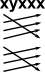(3 6 1)(2)(7 5 4)(8) (2 3 4 1)(6 7 8 5) (4 3 2 1)(8 7 6 5) (7 1)(6 2)(5 3)(8 4)

I don't know if these are the shortest sequences, perhaps I should get the program to check all combinations and see if these are any shorter sequences? At the moment the program allocates the name from the first case of a particular permutation it finds and then subsequent cases are given the original name.

So we don't need to use a third generator 'z' and we already have all 24 possible rotations.

Complete table:

 a*b b.i b.x b.y b.xx b.xy b.yx b.yy b.xxx b.xxy b.xyx b.xyy b.yxx b.yyx b.yyy b.xxxy b.xxyx b.xxyy b.xyxx b.xyyy b.yxxx b.yyyx b.xxxyx b.xyxxx b.xyyyx a.i i x y xx xy yx yy xxx xxy xyx xyy yxx yyx yyy xxxy xxyx xxyy xyxx xyyy yxxx yyyx xxxyx xyxxx xyyyx a.x x xx xy xxx xxy xyx xyy i xxxy xxyx xxyy xyxx yy xyyy y xxxyx yyx yyy yxx xyxxx xyyyx yx yyyx yxxx a.y y yx yy yxx xyx yyx yyy yxxx xx xyxx xxyx xxyy yyyx i xyyyx xxx xxy xyxxx xxxyx xyy x xxxy xy xyyy a.xx xx xxx xxy i xxxy xxyx xxyy x y xxxyx yyx yyy xyy yxx xy yx yy xyyy xyxx yyyx yxxx xyx xyyyx xyxxx a.xy xy xyx xyy xyxx xxyx yy xyyy xyxxx xxx yyy xxxyx yyx xyyyx x yxxx i xxxy yyyx yx xxyy xx y xxy yxx a.yx yx yxx xyx yxxx xx xyxx xxyx y xyyyx xxx xxy xyxxx yyy xxxyx yy xxxy yyyx i xxyy xy xyyy yyx x xyy a.yy yy yyx yyy xxyy xyxx yyyx i xyy yxx xyxxx xxx xxy x y xyyy yxxx xx xy xxxy xxyx yx xyyyx xyx xxxyx a.xxx xxx i xxxy x y xxxyx yyx xx xy yx yy xyyy xxyy xyxx xxy xyx xyy yxx yyy xyyyx xyxxx xxyx yxxx yyyx a.xxy xxy xxyx xxyy yyy xxxyx xyy yxx yyyx i xyyy yx yy yxxx xx xyxxx x y xyyyx xyx yyx xxx xy xxxy xyxx a.xyx xyx xyxx xxyx xyxxx xxx yyy xxxyx xy yxxx i xxxy yyyx xyyy yx xyy y xyyyx x yyx xxy yxx yy xx xxyy a.xyy xyy yy xyyy yyx yyy xyyyx x xxyy xyxx yyyx i xxxy xx xy yxx xyxxx xxx xxy y xxxyx xyx yxxx xxyx yx a.yxx yxx yxxx xx y xyyyx xxx xxy yx yy xxxy yyyx i xxyx xxyy xyx yyx yyy xxxyx xyxxx x xyy xyxx xyyy xy a.yyx yyx xxyy xyxx xyy yxx xyxxx xxx yy xyyy yxxx xx xy i xxxy yyy xyyyx x y xxy xyx xxxyx yyyx yx xxyx a.yyy yyy yyyx i xxy xyxxx x y xxyx xxyy xy yxxx xx yx yy xxxyx xyy yxx xyx xyyyx xxx yyx xyyy xyxx xxxy a.xxxy xxxy xxxyx yyx xyyy yx xxyy xyxx xyyyx x yxx xyx xyy xyxxx xxx yyyx xx xy yxxx xxyx yy i xxy y yyy a.xxyx xxyx yyy xxxyx yyyx i xyyy yx xxy xyxxx x y xyyyx yxx xyx xxyy xy yxxx xx yy xxxy xyxx xyy xxx yyx a.xxyy xxyy xyy yxx yy xyyy yxxx xx yyx yyy xyyyx x y xxx xxy xyxx yyyx i xxxy xy yx xxyx xyxxx xxxyx xyx a.xyxx xyxx xyxxx xxx xy yxxx i xxxy xyx xyy y xyyyx x xxxyx yyx xxyx yy xyyy yx yyyx xx xxyy yyy yxx xxy a.xyyy xyyy xyyyx x xxxy yyyx xx xy xxxyx yyx xxy xyxxx xxx xyx xyy yx xxyy xyxx xxyx yxxx i yy yxx yyy y a.yxxx yxxx y xyyyx yx yy xxxy yyyx yxx xyx yyx yyy xxxyx xxy xyxxx xx xyxx xxyx xxyy i xyyy xy xxx xyy x a.yyyx yyyx xxy xyxxx xxyx xxyy xy yxxx yyy xxxyx xyy yxx xyx y xyyyx i xyyy yx yy xx xyxx xxxy x yyx xxx a.xxxyx xxxyx xyyy yx xyyyx x yxx xyx xxxy yyyx xx xy yxxx xyxx xxyx yyx xxy xyxxx xxx xyy y yyy xxyy i yy a.xyxxx xyxxx xy yxxx xyx xyy y xyyyx xyxx xxyx yy xyyy yx xxxy yyyx xxx yyy xxxyx yyx x yxx xxy i xxyy xx a.xyyyx xyyyx xxxy yyyx xxxyx yyx xxy xyxxx xyyy yx xxyy xyxx xxyx xy yxxx x yxx xyx xyy xxx yyy y xx yy i

## Cayley Graph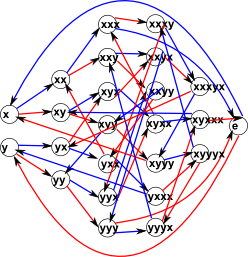## Specific Algebras to Represent this Group

metadata block
see also:

Correspondence about this page

Book Shop - Further reading.

Where I can, I have put links to Amazon for books that are relevant to the subject, click on the appropriate country flag to get more details of the book or to buy it from them.Fearless Symmetry - This book approaches symmetry from the point of view of number theory. It may not be for you if you are only interested in the geometrical aspects of symmetry such as rotation groups but if you are interested in subjects like modulo n numbers, Galois theory, Fermats last theorem, to name a few topics the chances are you will find this book interesting. It is written in a friendly style for a general audience but I did not find it dumbed down. I found a lot of new concepts to learn. It certainly gives a flavor of the complexity of the subject and some areas where maths is still being discovered.

Specific to this page here:

This site may have errors. Don't use for critical systems.

Copyright (c) 1998-2018 Martin John Baker - All rights reserved - privacy policy.